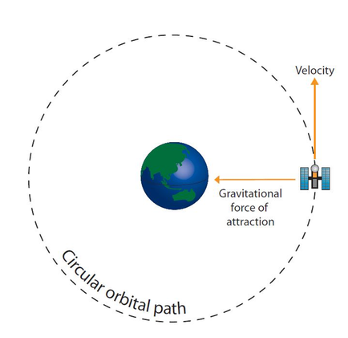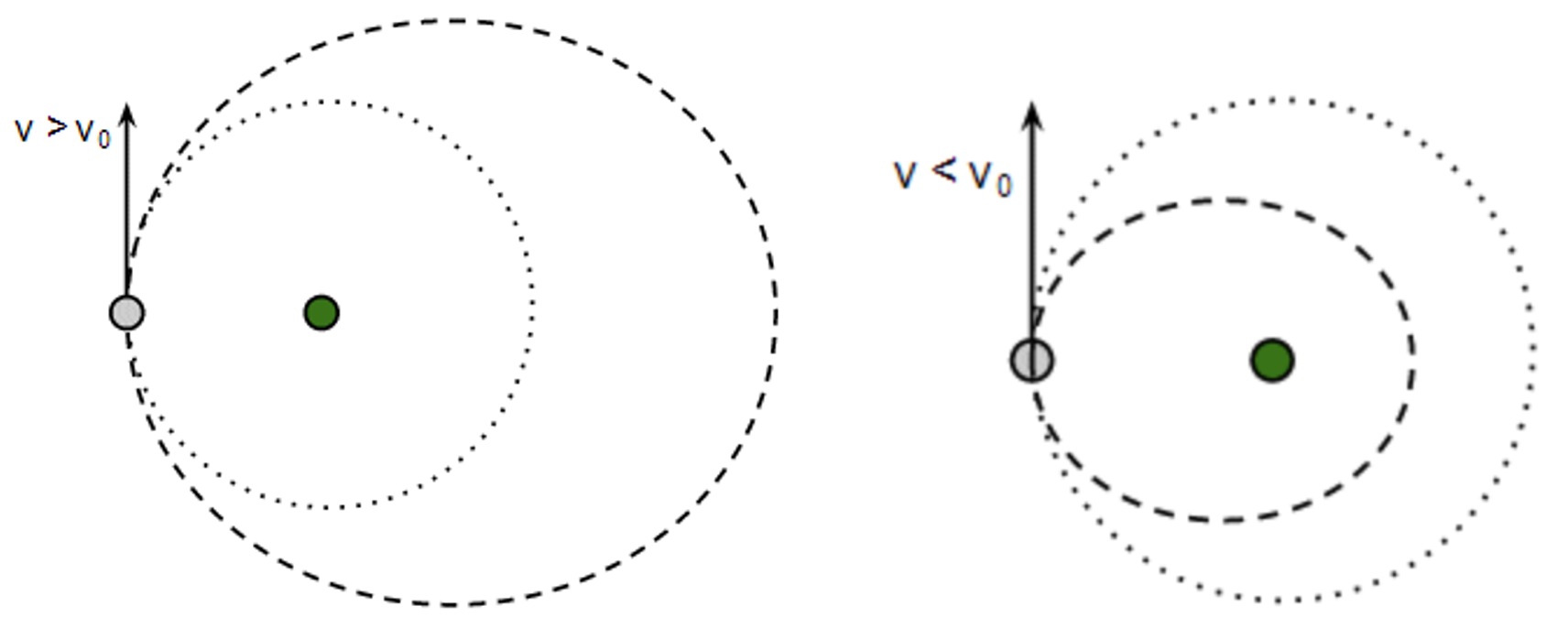# M5-S9: Newton's Law of Gravitation and Orbital Motion of Satellites

• ### Apply qualitatively and quantitatively Newton’s Law of Universal Gravitation to:

• determine the force of gravity between two objects

• investigate the factors that affect the gravitational field strength predict the gravitational field strength at any point in a gravitational field, including at the surface of a planet

Qualitatively: Newton’s Law of Universal Gravitation

There exists a force of attraction between any two masses of any distance. The magnitude of this force is directly proportional to the product of the two masses and inversely proportional to the square of their distance apart.

Quantitatively, this is expressed in the formula:

F_g=(GMm)/r^2

F is the gravitational force between two masses M & m (both in kg). G is the gravitational constant 6.67 x 10–11. r is the distance between the centre of the masses in metres (m).

The most important application of this law is the concept of gravity. Gravity arises from the gravitational attraction between Earth and an object in its gravitational field. Thus, when describing this force quantitatively, the masses are those of Earth and the object experiencing gravity respectively.

Additionally, by applying Newton’s second law F = ma, we can determine the acceleration an object is experiencing due to this force:

ma=(GMm)/r^2

a=(GM)/r^2

g=(GM)/r^2

Here, g is the gravitational acceleration which is independent of the object’s mass. On Earth, M refers to the mass of Earth. Given the following values:

• Mass of Earth: 6 x 1024 kg
• Average radius of Earth: 6371 km = 6 371 000 m

We can determine g:

g=((6.67xx10^(-11))(6.0xx10^24))/(6371000)^2

g=9.81 m s^(-2)

Gravitational acceleration is also referred to as the strength of gravitational field. These two terms are used interchangeably. There are several factors which influence the value of g.

• Distance from the earth’s centre – altitude
• Crust density
• Shape of the earth, equatorial diameters are greater than polar diameters, therefore they have lower acceleration due to gravity, while at the poles it has a stronger gravitational force

Practice Question 1
Discuss the following situations with regards to gravitational forcei. If the distance between two masses is doubled, find the change of the force.ii. If the mass of one object is doubled, find the change of the force.

Practice Question 2
Explain how the acceleration due to gravity varies according to the planet’s altitudes. (2 marks)

• ### investigate the orbital motion of planets and artificial satellites when applying the relationships between the following quantities:

• gravitational force
• centripetal force
• centripetal acceleration
• mass
• orbital velocity
• orbital period

Orbital Motion of Planets and Artificial Satellites

In space, a satellite experiences gravitational force exerted by Earth. The direction of this force is pointing towards the centre of Earth. In essence, when a satellite is orbiting around Earth due to gravity, the centripetal force (circular motion) is strictly caused by gravitational force.

F_c=F_g

(mv^2)/r=(GMm)/r^2

v^2=(GM)/r

v_(orbital)=sqrt((GM)/r)

By rearranging the equation (see above), we can determine the velocity needed for the satellite to achieve this circular motion. This is called the orbital velocity as circular motion in this context is simply orbiting around Earth. It is important to understand the relationship between orbital velocity, mass of planet a satellite is orbiting around and the radius of orbit.Forces acting on an object in orbit: objects e.g. satellites primarily experience gravitational force during orbit. Since the centripetal force is caused by gravitational force, we cannot state centripetal force as the real cause of motion.

If a satellite can achieve and maintain a certain orbital velocity (dependent on the planet’s mass and radius of orbit), it can stay in orbit without additional force nor acceleration in the same direction as its motion. In other words, it is possible to undergo stable orbit with only gravitational force. Travelling at a velocity greater than the orbital velocity will cause the satellite to take on a different orbit path.

Orbital period is defined as the time taken for an object to complete one revolution of an orbit. The orbital period is shorter when the circumference or radius of orbit is smaller. Vice versa.Practice Question 3

A satellite, with a mass of 200 kg, orbits in a circular motion around the Earth at an altitude of 300 km.

(a) What is the centripetal force acting on the satellite?

(b) What is the orbital velocity of the satellite?

Practice Question 4

A satellite has a mass of 250 kg and orbits the Earth at an altitude of 3000 km.

(a) Determine its orbital speed.

(b) Compare the orbital speed of a 500 kg satellite in an identical orbit.

Practice Question 5

The average orbital separation between surface of Earth and surface of Sun is 1.5 × 1011 m.Using the data from the table above, determine the orbital speed of the Earth. (2 marks)

Next section: Kepler's Laws of Planetary Motion